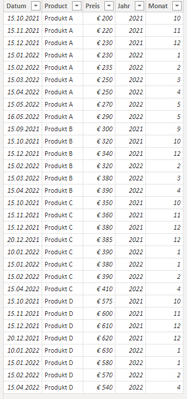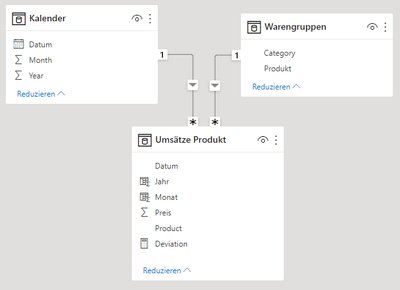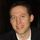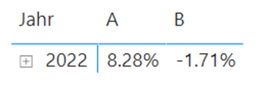cancel
Showing results for
Did you mean:Frequent Visitor

## Average of a Measure per Category

Hey guys,

I have 3 simplified data tables as follows (the third is a calendar table):

"Umsätze Produkt""Warengruppen"First I wanted to calculate the deviation of the product prices (latest price last year compared with the max prices per month from this year).

This results in the table as follows with the help of you guys in another thread:The Deviation is calculated by following code:

``````Deviation =
VAR CurrentPrice =
CALCULATE (
MAX ( 'Umsätze Produkt'[Preis] ),
'Umsätze Produkt'[Jahr] = YEAR ( TODAY () )
)
VAR LatestPrice =
CALCULATE (
LASTNONBLANKVALUE ( 'Umsätze Produkt'[Datum], SUM ( 'Umsätze Produkt'[Preis] ) ),
PREVIOUSYEAR ( Kalender[Datum] )
)
RETURN
IF (
DIVIDE ( CurrentPrice, LatestPrice ) - 1 = -1.00,
BLANK (),
DIVIDE ( CurrentPrice, LatestPrice ) - 1
)``````

The final result I need is the average deviation per category broken down per month:As you can see, it's empty and I really don't know how to calculate the average of a measure...

Could you guys help me out?

Thx and greets

1 ACCEPTED SOLUTIONSuper User

Thanks for the file. I rewrote the measure to include additional columns in the virtual table (in case they aren't in the visual):

``````Deviation Average =
VAR vTable =
SUMMARIZE (
'Umsätze Produkt',
Kalender[Year],
Kalender[Month],
'Umsätze Produkt'[Product],
Warengruppen[Category]
),
"@Amount", [Deviation]
)
RETURN
AVERAGEX ( vTable, [@Amount] )``````Is this correct? If not, can you provide a screenshot of the expected result?

Proud to be a Super User!

6 REPLIES 6Super User

You could try a measure like this:

``````Deviation Average =
VAR vTable =
ADDCOLUMNS ( VALUES ( Warengruppen[Category] ), "@Amount", [Deviation] )
VAR vResult =
AVERAGEX ( vTable, [@Amount] )
RETURN
vResult
``````

Proud to be a Super User!Frequent Visitor

I tried this measure and get this result:When I put in the upper table it shows the exact same values but it doesn't aggregate in the table below. Is there something wrong with my implementation?

GreetsSuper User

I would need to see your pbix. Can you share a sanitized version via one of the file services like OneDrive?

Proud to be a Super User!Frequent Visitor

I found several errors.

• It only shows some values of the "Deviation Average" when the colums Year and Month are included in the table
• The Average Deviations per month are too low. I think it includes the months without a price because it results in -100% (see the Measure "See Error"). I tried to exclude them in the Measure "Deviation" but apparently it doesn't work.
• The matrix "Category-Month-Average Deviation" doesn't work completely...

How can we exclude the -100% and visualize the Average Deviations per months correctly?

Thank you for your help. Much appreciated.Super User

Thanks for the file. I rewrote the measure to include additional columns in the virtual table (in case they aren't in the visual):

``````Deviation Average =
VAR vTable =
SUMMARIZE (
'Umsätze Produkt',
Kalender[Year],
Kalender[Month],
'Umsätze Produkt'[Product],
Warengruppen[Category]
),
"@Amount", [Deviation]
)
RETURN
AVERAGEX ( vTable, [@Amount] )``````Is this correct? If not, can you provide a screenshot of the expected result?

Proud to be a Super User!Frequent Visitor

Absolutely perfect!

Thank you!